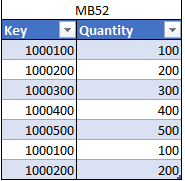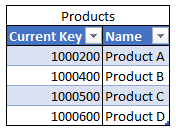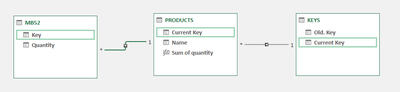cancel
Showing results for
Did you mean:Frequent Visitor

## Measure to sum a column bases on another tables column

Hello guys, it's my first post here and I'm very glad to be part of this forum.

Recently I'm trying to deal with a logical sum in DAX and to be honest I not dealing with it really well...

My problem is:

I have 3 tables:I want to sum the MB52[Quantity] based on Current and Old Keys, reaching this result:Identifying [Current Key] and suming the quantity of the [Old. Key] with owns [Current Key] quantity.

I tried this code but with no sucess 😞

`[Sum. Quantity] =IF('KEYS'[Old. Key] <> "";CALCULATE(SUM(MB52[Quantity]) ; MAX(MB52[Key]) = MAX('KEYS'[Old. Key])) + CALCULATE(SUM(MB52[Quantity]) ; MAX(MB52[Key]) = MAX('KEYS'[Current Key]));SUM(MB52[Quantity]))`

I apreciate a lot your help and thanks anyway 🙂

1 ACCEPTED SOLUTIONCommunity Support

Here are the steps you can follow：

1. Create measure.

``````Measure =
var _old=
CALCULATE(SUM('MB52'[Quantity]),
FILTER(ALL(MB52),
'MB52'[Key]=MAX('Keys'[Old.Key])))
var _current=
CALCULATE(SUM('MB52'[Quantity]),
FILTER(ALL(MB52),
'MB52'[Key]=MAX('Keys'[Current Key])))
var _sum=
_old + _current
return
IF(
_sum = BLANK() ,0,_sum)``````
``````Measure 2 =
SUMX(
FILTER(ALL('Keys'),
'Keys'[Current Key] = MAX('Products'[Currrent Key])),
[Measure])``````

2. Result:Best Regards,

Liu Yang

If this post helps, then please consider Accept it as the solution to help the other members find it more quickly

4 REPLIES 4Community Support

Here are the steps you can follow：

1. Create measure.

``````Measure =
var _old=
CALCULATE(SUM('MB52'[Quantity]),
FILTER(ALL(MB52),
'MB52'[Key]=MAX('Keys'[Old.Key])))
var _current=
CALCULATE(SUM('MB52'[Quantity]),
FILTER(ALL(MB52),
'MB52'[Key]=MAX('Keys'[Current Key])))
var _sum=
_old + _current
return
IF(
_sum = BLANK() ,0,_sum)``````
``````Measure 2 =
SUMX(
FILTER(ALL('Keys'),
'Keys'[Current Key] = MAX('Products'[Currrent Key])),
[Measure])``````

2. Result:Best Regards,

Liu Yang

If this post helps, then please consider Accept it as the solution to help the other members find it more quicklyFrequent Visitor

It is what I was trying to do!

Thank you so much @v-yangliu-msft, you helped me to reach the result and learn a lot about DAX with this problem!Frequent Visitor

Sorry, I forgot to post the tables relationship...Because I'm using power pivot I had to do some changes, using INTERSECT instead TREATAS and use DISTINCT on MB52[Key].

The final result is just a sum of Current Keys quantity and putting the Old Keys quantity in a null key 😞

`Sum of quantity = CALCULATE(                 SUM ( MB52[Quantity] );                  INTERSECT(                          UNION( VALUES ( KEYS[Current Key] ); VALUES( KEYS[Old. Key] ) );                          DISTINCT(MB52[Key])                )           )`

Result:Super User

Assuming that you have a one-to-many relationship from Products to Keys and no relationships between MB52 and the other tables, then you could try

``````Sum of quantity =
CALCULATE (
SUM ( MB52[Quantity] ),
TREATAS (
UNION ( VALUES ( Keys[Current Key] ), VALUES ( Keys[Old Key] ) ),
MB52[Key]
)
)``````